Unlimited Plugins, WordPress themes, videos & courses! Unlimited asset downloads! From \$16.50/m# Simulate Projectile Motion with ActionScript 3.0

This post is part of a series called You Do The Math.
Quick Tip: Trigonometry for Flash Game Developers
Creating Generative Art with HYPE

In this tutorial I’m going to take you through the process of simulating basic projectile motion using Flash and ActionScript. Our simulation will dynamically animate the initial trajectory of a projectile, and its subsequent bounces against the ground.

## The Final Result

Here's a quick preview of what we'll be working towards:

## Introduction

One of the elements that makes modern video games so realistic is their physics simulation. By applying concepts of classical mechanics to our Flash animations, we can create stunning visual effects that enhance the overall user experience.

In this tutorial I'm going to take you through the process of simulating basic projectile motion using Flash and ActionScript. Our simulation will dynamically animate the initial trajectory of a projectile, and its subsequent bounces against the ground. To keep things simple I have not included air resistance, so the example projectile will only experience one force; gravity. This tutorial assumes you have some basic knowledge of object-oriented programming and ActionScript. You may also benefit from having some experience with quadratic functions, and basic concepts of motion like acceleration and velocity. Although I am mostly going to be covering the application of these concepts as they apply to Flash and ActionScript, I want to first take some time to briefly explain them as they apply to classical mechanics.

Classical mechanics is the branch of physics based on Newton's laws of motion. In this tutorial we're going to look at two concepts of classical mechanics: projectile motion, and the coefficient of restitution. Projectile motion describes the path (trajectory) of an object with an initial velocity, while experiencing acceleration from gravity (and in most cases air resistance). You're probably familiar with the story of Newton formulating his theory of gravity while sitting under an apple tree. Gravity plays a big role in projectile motion, since it adds a constant acceleration of -9.8 m/s2 in the y-direction.

A good example of projectile motion is a baseball being thrown in the air. When you throw a baseball, it has an initial speed and direction at the instant it leaves your hand. These two properties make up the ball's initial velocity. After the ball leaves your hand, it follows an arc shaped path, also known as its trajectory. The general trajectory of a projectile can be described by a quadratic function: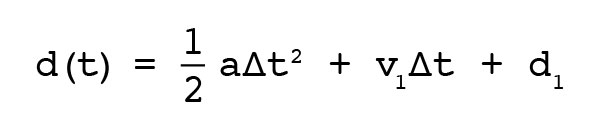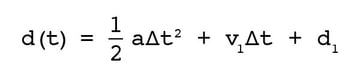This function gives us the position of a projectile with respect to time (d(t) is the position after t seconds have passed). The little triangle means "change in", so Δt means "number of seconds passed".

To use this function we need to know:

• The acceleration, a, of the projectile;
• The initial velocity (speed in a particular direction), v1, of the projectile;
• The initial position, d1, of the projectile.

The path of a projectile in the x and y directions are independent. This means that in order to calculate the position in a 2-dimensional space, we need to use the function twice.

The other concept we're going to be looking at is something called the coefficient of restitution. The coefficient of restitution is a ratio for describing the difference in an object's velocity before and after a collision.

For example, if you bounce a basketball against the floor, the height it bounces decreases with each subsequent bounce. This change in height (directly related to the change in velocity), is constant, and can therefore be described by the coefficient of restitution. Calculating the coefficient of restitution for a moving object and a stationary surface is simple: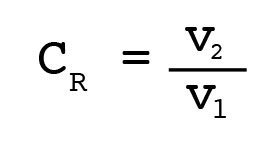Here, v1 is the velocity of the ball just before it hits the ground, and v2 is the velocity of the ball just after it hits the ground and starts bouncing upwards again.

Since the coefficient of restitution is different for every combination of objects, you will need to find your own, depending on what you are simulating.

## Step 1: Setting up the FLA Document

Let's start building this thing! Open up Flash, and create a new document. In the example I will be using the default dimensions of 550 x 400, and a frame rate of 50 FPS. Save this file under a name of your choice.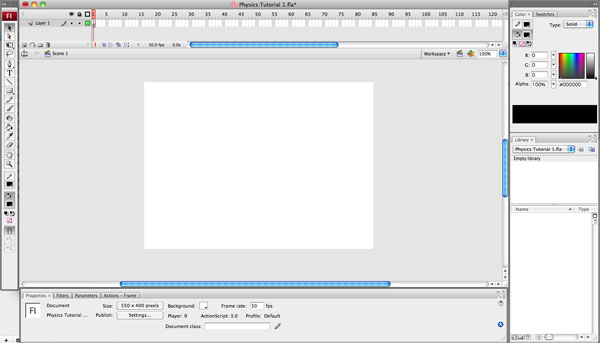Next, we need to create a document class. Create a new Actionscript file, and add the following:

Save this file in the same directory as our FLA. Call it Main.as.

The last thing we need to do is link the document class with the FLA. Inside the FLA, find the Properties panel. Next to Document class, enter the name of the document class, Main.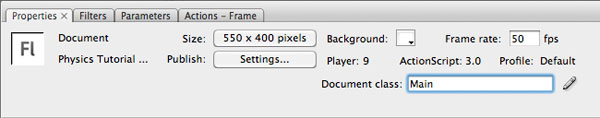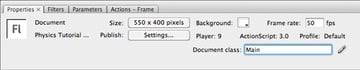## Step 2: Creating the Main Loop

Now that our flash document is set up, we can start coding. The first thing we need to do is create a loop that will run at a fixed time interval. Generally speaking, there are two trains of thought regarding timed loops: using the ENTER_FRAME event, or using the AS3 Timer class. Both methods have different pros and cons, but for this example I am going to use the Timer class.

We need to start by importing two classes: the Timer class, and its respective TimerEvent class. Inside Main.as add the following lines.

Before we set up the Timer, I want to add another method called, mainLoop to the document class. As its name suggests, this method will serve as our loop.

Now we can set up the Timer! We're going to start by adding two properties to the class: masterTimer, and interval. The masterTimer's purpose should be obvious, but the interval property will be used to store the length (in seconds) of a Timer interval.

Next we're going to populate these properties inside our constructor method, Main. In Step 1 we set our document's frame rate to 50 FPS. This frame rate can be referenced with stage.frameRate, and will be used as the basis of calculating the Timer interval.

The Timer generates a TimerEvent.TIMER event every interval (in our case, 50 times a second, or once every 20 milliseconds). By adding an event listener to the Timer, we can run our mainLoop method every time this event is generated, thus creating our loop.

The only thing remaining is to start the Timer.

## Step 3: Testing the Main Loop

If we test the movie as is, nothing will happen. Since we want to know if the loop is working, we are going to add a line to the mainLoop method that outputs the amount of time the SWF has been running. By default the Timer can only return the current number of intervals that have passed. Since we already calculated the length of an interval, we simply multiply the two values to get the time. Please note that I am rounding the result to avoid issues with floating point arithmetic.

If we trace currentTime, we can see the Timer working.

Test the movie. Your output log should look similar to this: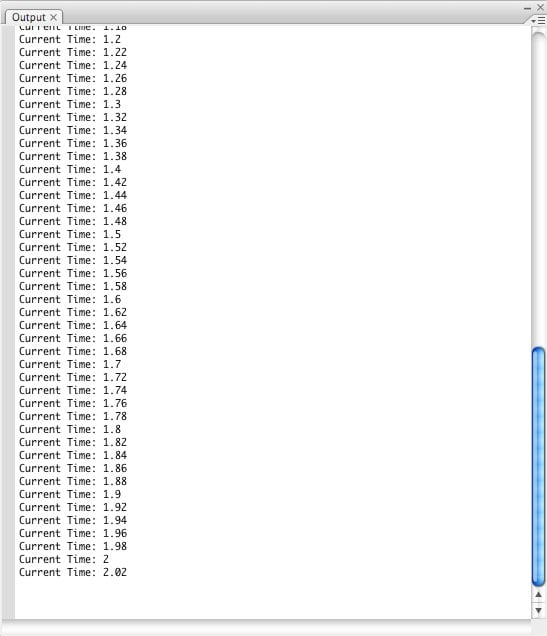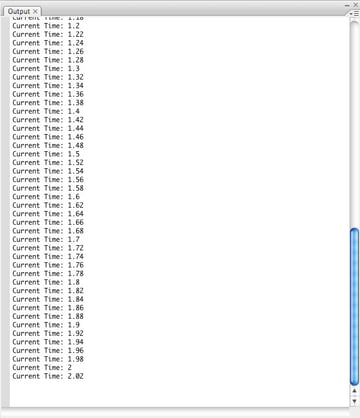Since we know the Timer is working, we can remove the trace statement.

## Step 4: Projectile.as Creating the Class

Our next step is to create a class that represents a projectile (the basketball). Create another ActionScript file, and once again add our basic class structure. Save this file as Projectile.as.

We're going to start by adding some properties to keep track of the projectile's characteristics. I will explain the animation specific properties later. The rest you should recognize from the equations at the start of the tutorial.

## Step 5: Projectile.as Convenience Methods

Since this project uses a lot of calculations, it makes sense to write a set of convenience methods for the tedious calculations which will be performed numerous times. Below are six convenience methods that will be referenced throughout the rest of the tutorial.

The first method, solveQuadratic, return the roots of a quadratic function (in standard form: Ax2 + Bx + C; this method will return the value of x) using the quadratic formula.

The second method, getComponents, returns an array with the individual x and y components of a Euclidean vector.

The third method, getPosition, will return the position at a given time. We're using the position formula from the introduction; this is d(t).

The fourth method, getTimes, is the opposite of getPosition. It returns the time taken for the projectile to reach a given position.

The fifth method, getVelocity, uses the first derivative of our position function to return the velocity of the projectile at a given time.

The sixth method, getVelocityDirection, returns the direction (angle) of the velocity at a given time. In other words, it returns the direction that the projectile is moving in at a specific instant.

## Step 6: Projectile.as Initialization Method

In step 4, we added a bunch of properties to the Projectile class. If you noticed, all these properties are private. I did this because we don't want to access any of them directly. We still need a way to set and preform the necessary calculations on these properties, so we're going to add an init method to do so.

The names of the different parameters should be fairly obvious. The velocityDirection parameter takes the angle of the initialVelocity in degrees. The acceleration parameters are all optional. If you set a value for acceleration, you will need to provide a separate value for accelerationDirection as we did with velocityDirection.

I mentioned earlier that we're dealing with components, however our init method only accepts a vector (magnitude and direction). This is where our convenience methods come into play.

Now we have an array containing the individual components of the vectors. We can now store them to their corresponding properties.

The points for initial position do not need to be changed.

## Step 7: Projectile.as Control Methods

Before we continue, I need to explain the animation-specific properties we created in Step 4. I'll start with the moving property. Since our projectile has a rest state, we use the moving property as a flag so the loop only animates when the projectile is in motion. The startTime property is used to account for the difference between the global time of the Timer, and the local time of the projectile's position function. Nothing too complicated.

Our control methods, for lack of a better term, will, for the most part, be called from within the loop. These first three methods are really simple, and are used to interact with the animation-specific properties.

The last method we're going to add deserves most of our attention. As its name suggests, the positionAtTime method returns the position of the projectile at a given time. We can use this position inside the loop to animate our MovieClip on the stage.

If you look inside this method, you should notice a couple of things. This first thing is the use of a relativeTime variable. I mentioned earlier that we need to account for the difference in time between the Timer and Projectile. The beginning of the projectile's trajectory starts at a relative time of 0, so we're only interested in the the amount of time that has passed on the Timer since this trajectory began. This is why we store the startTime. The second thing is the use of our getPosition method from step 5. We use this method twice to calculate the position of the Projectile in both axes.

Save the Projectile class.

## Step 8: Projectile.as Linking to a MovieClip

Before we can animate, we need something to animate. If you noticed, our Projectile class extends the MovieClip class, which means we can link it to a MovieClip in our library.

Open the main flash document (the FLA), and draw a circle on the stage. Don't worry too much about the size, since we're going to scale it down later in code.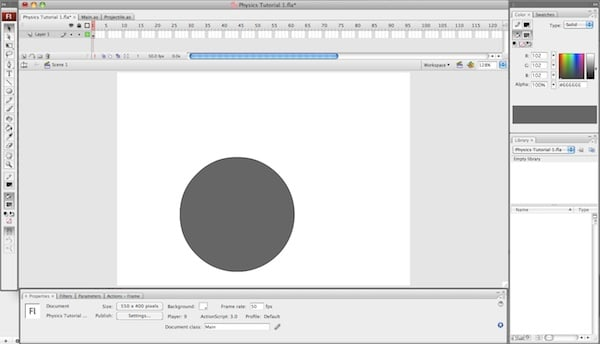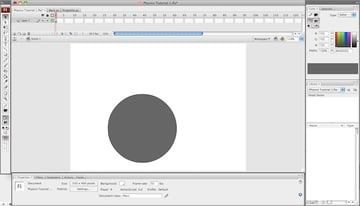Next, convert the circle to a MovieClip. The MovieClip should appear in your library, once it does, delete it from the stage since we will be adding it programmatically in the next step.

Now we need to link our Projectile class with the MovieClip. In your library right click (Control click on the Mac) your MovieClip and select Linkage...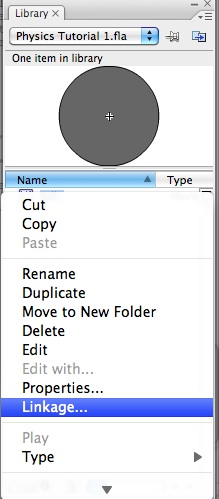Check the box beside Export for ActionScript. Enter Ball for Class, and Projectile for Base class.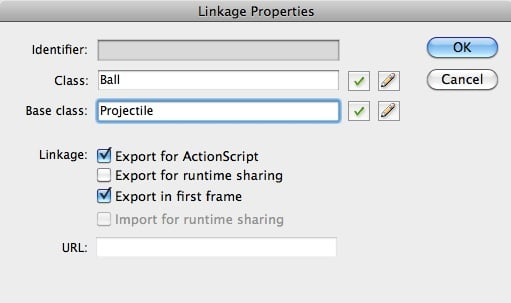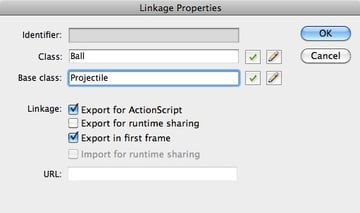Click OK. You may see a dialogue box telling you that a class definition could not be found, and that one will be generated automatically. Click OK again.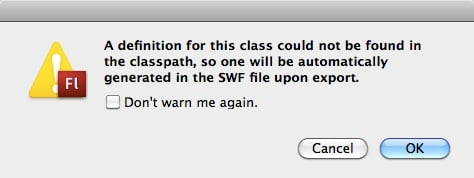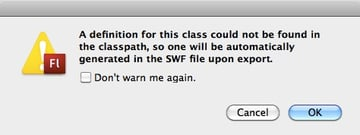Finally, save the document.

## Step 9: Animating!

We have a loop, we've written our Projectile class, and we've linked it with a MovieClip. Now it's time to animate the projectile! To begin, open our document class, Main.as.

We're gonna start by adding a few more properties.

The purpose of these properties is pretty straightforward. The scale property is going to represent a scale between Flash's pixel position and our calculated metre position. The ball property represents the MovieClip we created in the previous step. You might notice that its type is set to Ball, this is the class name we set in the previous step. Finally the startFlag property is used as a flag to tell the loop when our projectile should begin its trajectory.

Inside the constructor method, we're going to add the following lines to setup our Ball object.

If you test the movie, your ball should appear in the bottom-left corner of the stage.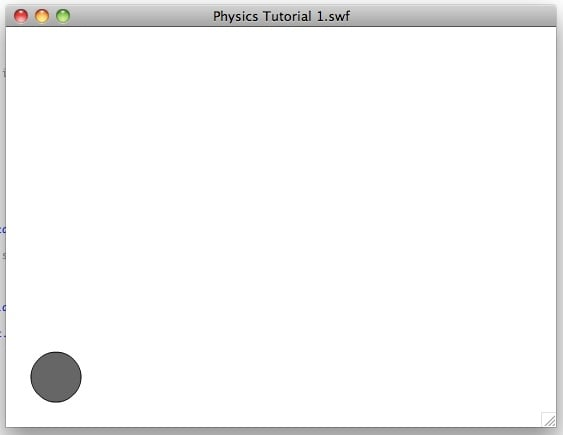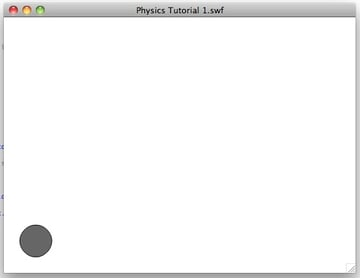Next we need to run the init method from step 6. If you look at the method declaration, you'll see that it requires a minimum of 4 paramters: velocityDirection, initialVelocity, initialPositionX, and initialPositionY. Staying in the constructor method, let's set those up!

The parameters for initial position require some simple calculations before they're passed on to the method. The Projectile methods all use metres as the unit of distance, so we need to use our scale. The initialPositionY requires an additional step. Since Flash's origin exists in the top-left corner, and increasing y moves down the screen, we need to subtract our y-position from the stage.stageHeight, to move our origin to the bottom-left and allow increasing y to move up the screen.

Now that our projectile is configured, we can set the startFlag.

Let's move on to setting up the loop. The first thing we need to do is add a conditional statement that checks for the startFlag.

There's a few things worth noting. If you recall, we already created a variable called currentTime in Step 3. Also, since we only want to call the begin method once, we set the startFlag property back to false.

It would be pointless for the loop to calculate the position of the projectile if it's at rest, so we need to add another conditional statement that checks whether the projectile is moving.

Inside this statement we can implement the actual animation. The first thing we need to do is get the projectile's position.

Once we know the position, we simply update the MovieClip's position. Similar to the init method, we need to take into account our scale, and Flash's origin.

We've finally reached a milestone! If you test the movie now, you can see the basic effect!

## Step 10: Making the Ball Bounce

We've animated the trajectory of our projectile, but as you can see the ball keeps moving indefinitely. To enhance this simulation, we're going to create a ground on which the ball can bounce. For simplicity's sake, we're going to make our ground an imaginary line, at the bottom of the stage.

Our approach here involves implementing collision detection. Generally speaking we have two options for our collision detection: frame-based and predictive. Ultimately we are going to be using predicative collision detection, but I think its worth explaining the theory behind each option:

The idea behind frame-based collision detection is simple. Inside the loop, we would check whether the ball's y-position is below the ground's y-position. If the condition is met, a collision has taken place...sounds good right? Not really. Chances are that a collision will not happen on an exact Timer interval. This means that the time and position of the bounce will be inaccurate. Consider this diagram: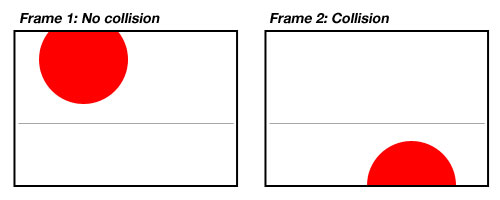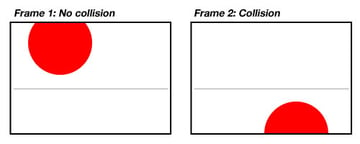The actual collision would have taken place in between the two frames. But our frame-based collision detection code would only have detected the collision in the second frame, when the projectile should already be on its new trajectory.

We can overcome this problem by using predictive collision detection. It can be slightly more challenging to implement, but it gives us a more accurate simulation. Predictive collision detection works as follows. We first calculate, in advance, the exact time that the projectile will collide with the ground. Inside the loop we check whether this time has passed. If it has, we set the projectile on a new trajectory, starting at that exact time of collision.

First thing's first, we need to add a few more methods to our Projectile class. We'll start with timeOfCollision.

(Remember, getTimes returns the time at which the projectile will be at a specified position. It will actually return two times, one negative and one positive, because these are the roots of a quadratic equation.)

We're also going to need to know the magnitude and direction of our velocity at this time, so add these two methods for calculating that as well.

Before we finish up with this class, I want to add one more method called getStartTime. We will be using it in the next step.

Save the Projectile class and return to the document class. The first thing we need to do is add a couple of properties. A collisionTime property, and a cor property to represent our coefficient of restitution.

The first thing we need to do is set our cor. We can set this in the constructor method. I've picked 0.8, but feel free to experiment with different values to see the effects.

Now for the fun part! Inside our main loop, we need to implement our bouncing methods. For starters, find the condition for startFlag. Inside add the following line. This will calculate our initial collision time.

Next find the condition that checks ball.isMoving(). We're only going to check for collisions if the ball is in motion, so we'll put our collision detection statements in there. Add the following to the top of the condition.

Inside that collision condition, we need to execute the bounce. We achieve this by setting the projectile on a new trajectory, starting at collisionTime. The first thing we need to do is calculate the direction and magnitude of the velocity at collisionTime.

Next we need to run the appropriate methods for setting the new trajectory. For the timeOfCollision method, we need to set our ground y-position to half the height of the ball. We do this because the y-position of the ball is actually at its centre point, not its base.

We've reached another milestone! If you test the movie, you should see the bouncing effect.

## Step 11: But the Bouncing Never Stops!

If you take a look at the above example, the ball never comes to a complete stop. Towards the end it appears to have a quick, jittery motion. This phenomenon is caused by the following line:

I'll explain. Assuming cor is not equal to 0 or 1, our projectile's velocity will only approach zero, getting slower and slower, but never actually reaching it. Since the reality is that our projectile does come to rest, we need to implement a solution for this problem.

Here's how. Since the cor is constant, we can look at the bounces as a geometric series:

• a1: Time taken for first bounce
• a2: Time taken for second bounce
• a3: Time taken for third bounce
• ...

Each a term in the sequence represents the length of time for a bounce. We then refer to the cumulative time taken like so:

• S1: Time taken for first bounce
• S2: Time taken for first bounce and second bounce
• S3: Time taken for the three first bounces, in total
• ...

From here, we can use the formula for the sum of an infinite geometric series to calculate our stopping time: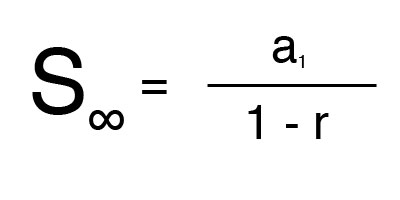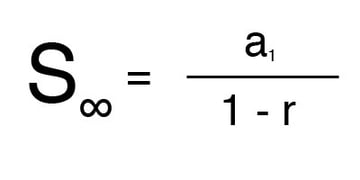The formula itself is simple. The a1 variable, represents the first term in the series, in our case, the length of the first bounce. The r variable represents the series' ratio, in our case, the cor.

As far as the implementation goes, we'll start by adding two more properties to the document class.

We need to know when the first bounce occurs, so the bounce property will be used as a simple counter. Inside the loop's startFlag condition (this if-statement: if(startFlag == true){) add the following after our declaration of cor:

Next, add the following to the end of our collision condition.

Every time a collision occurs we need to check whether stoppingTime has passed. If it has we can bring the projectile to rest, by calling the end method. The loop is still going to reposition the ball one last time, so we need to set the ball to its correct final position. The loop already set the projectile on a new trajectory, so we're going to get around this by setting currentTime to ball.getStartTime().

That's it! The projectile now bounces and comes to a complete stop.

## Step 12: Adding Basic UI Control

When we play the movie, the projectile fires immediately. This is pretty useless for most applications, so let's change things a bit and add some basic UI controls. To keep things simple, we're going to add two buttons: a start button, and a reset button.

Our first step is similar to Step 8. In the FLA document, draw two buttons for Start and Reset. Convert each button to a symbol, this time make them Buttons as opposed to MovieClips. This time we are going to keep our buttons on the stage, so position them as desired. Similarly to Step 8, open the Linkage Properties for one of the buttons. Check the box beside Export for ActionScript, this time however, leave the default class names. Click OK and repeat this for the other button.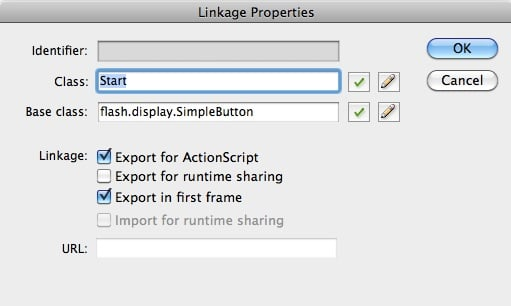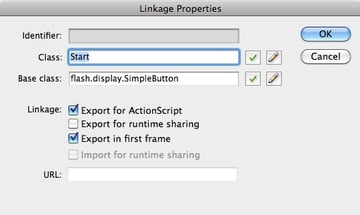On the stage, select the Start button. Open up the Properties panel. Where it says <Instance Name> enter startButton. Repeat this for the reset button, calling it resetButton.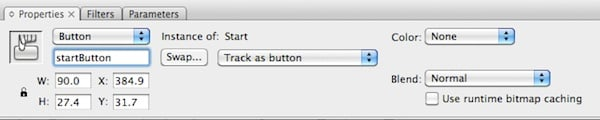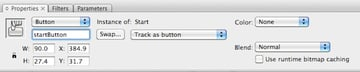Save this file, and open the document class. Inside, we need to import the MouseEvent class.

Inside the constructor method remove the following lines.

We're going to replace this by adding event listeners for each of the buttons we created. Notice that we can reference them with the <Instance Name> we set earlier.

Finally, we can wrap everything up by adding the two methods we're calling from those event listeners.

## Conclusion

Although the final result is simple, it works, and it shows how easy it is to implement real, dynamic physics concepts into your flash projects. How you choose to apply them comes down to your own creativity. If you're looking for a more complete, intuitive solution, it might make more sense to check out an existing physics engine like QuickBox2D.

To summarize: we've created a timed loop, written a class that models the trajectory of a projectile and added the necessary collision detection to simulate bounce. We covered a lot of content, but ultimately, we've barely scratched the surface of what we can do with classical mechanics. In projectile motion alone, there is still room to add things like drag, air resistance, and terminal velocity.

I hope you have enjoyed this tutorial. Thanks for reading!